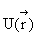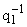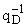H. S. Babajanyan, S. A. Babajanyan

Bose-Einstein Condensation of the D-dimensional System of the Ideal Bose-gas
with Excitation Spectrum
ep = cps in the Presence of Trapping Potential with
an Arbitrary ProfileAsymptotical methods are used to derive the criterion of BEC possibility, and the criteria determining the order of phase transition at BEC and indicating that BEC can be a phase transition (PTn) of an arbitrary order v О [2,Ґ) with finite or infinite jumps of the k-th (k = n - 1) derivatives of chemical potential m(T) and total energy E(T) with regard to T and that all this is being determined by parameter c = D/s++ј+(qi - are the exponents of calibrating functions of asymptotical behavior ofat the minimum point). Parameter c takes on an infinite sequence of values (ck = k/(k - 1)), setting the Dc interval in which the BEC is possible, and for each PTn Dcn subintervals of Dc and on each Dcn there are subintervals where the jumps of m(T) and E(T) are either finite or infinite. The universal role of parameter c in systematization of infinite variety of extended systems "external field-system of bosons" by classes of identical nature of phase transitions at BEC is also shown.